Next: Dimensionless Numbers in Incompressible Up: Mathematical Models of Fluid Previous: Equations of Incompressible Fluid

# Equations of Compressible Fluid Flow

In many situations of general interest, the flow of gases is compressible. In other words, there are significant changes in the mass density as the gas flows from place to place. For the case of compressible flow, the continuity equation (1.40), and the Navier-Stokes equation (1.56), must be augmented by the energy conservation equation (1.75), as well as thermodynamic relations that specify the internal energy per unit mass, and the temperature in terms of the density and pressure. For an ideal gas, these relations take the form (Reif 1965)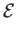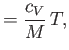(1.83)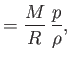(1.84)

where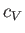is the molar specific heat at constant volume,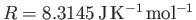the molar ideal gas constant,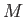the molar mass (i.e., the mass of 1 mole of gas molecules), andthe temperature in degrees Kelvin. Incidentally, 1 mole corresponds to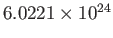molecules. Here, we have assumed, for the sake of simplicity, thatis a uniform constant. It is also convenient to assume that the thermal conductivity,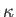, is a uniform constant. Making use of these approximations, Equations (1.40), (1.75), (1.83), and (1.84) can be combined to give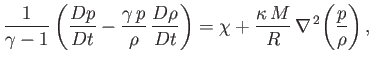(1.85)

where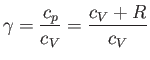(1.86)

is the ratio of the molar specific heat at constant pressure,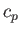, to that at constant volume,. [Incidentally, the result that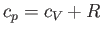for an ideal gas is a standard theorem of thermodynamics (Reif 1965).] (See Section 14.2.) The ratio of specific heats of dry air at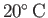is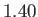(Batchelor 2000).

The complete set of equations governing compressible ideal gas flow are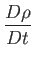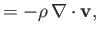(1.87)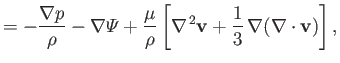(1.88)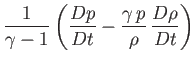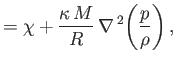(1.89)

where the dissipation function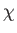is specified in terms of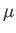and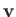in Equation (1.74). Here,,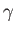,,, and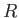are regarded as known constants, and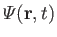as a known function. Thus, we have five equations--namely, Equations (1.87) and (1.89), plus the three components of Equation (1.88)--for five unknowns--namely, the density,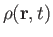, the pressure,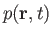, and the three components of the velocity,.Next: Dimensionless Numbers in Incompressible Up: Mathematical Models of Fluid Previous: Equations of Incompressible Fluid
Richard Fitzpatrick 2016-03-31